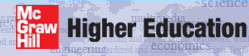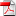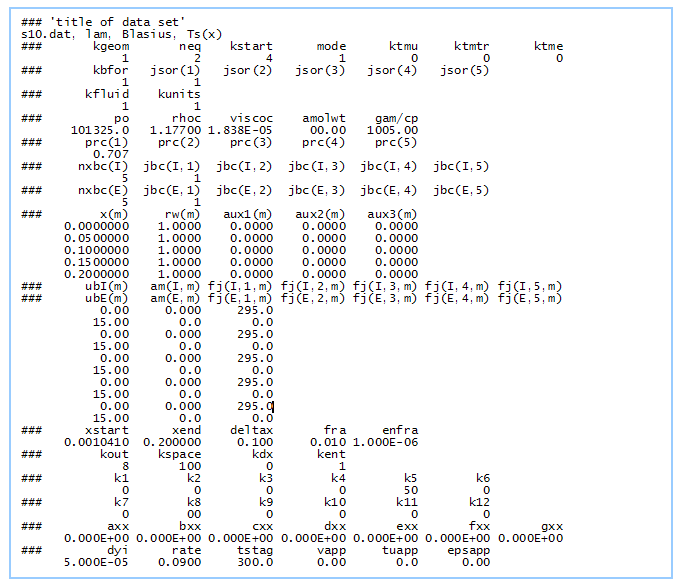# Institut für Thermodynamik der Luft- und Raumfahrt - Universität StuttgartMechanical Engineering - The University of Texas at Austin# setup and run TEXSTAN

The block Starting TEXSTAN presents what TEXSTAN looks like in the Microsoft DOS Window when TEXSTAN is executed. Building a TEXSTAN Dataset presents a description of the input variables that are used to build the dataset, and TEXSTAN Output Format Choices presents an idea of what to expect for TEXSTAN output and possible choices in data presentation formats.

Starting TEXSTAN - When the Windows-compiled TEXSTAN is started, a DOS window opens, and you are interactively prompted for the name of the input file name.If you type in a file name and this file does not exist (maybe you misspelled the name or left off the ".txt" extension), it reprompts for the name. If you decide to abort TEXSTAN rather than typing in an input dataset name, you may "kill" the start by mouse-clicking the "x" in the upper right-hand corner of the DOS window.

To execute a data file (in this example s10.dat.txt) type in the dataset name and execution begins.The first output file in.txt is opened for writing, and as TEXSTAN reads each line of the input dataset it does input error checking for formatting errors and data consistency, and then it writes the data line to the in.txt file. At any time during the reading of the input dataset TEXSTAN may stop, declaring an input dataset error and (hopefully) a hint as to the problem. As soon as the input dataset is successfully read and accepted, TEXSTAN closes the in.txt file.

Once the input dataset has been successfully processed, TEXSTAN opens the output file out.txt, followed by a write to the DOS window of the first line of the input dataset (the title variable). At this point integration begins and any output that is generated is written to this file. To monitor the status of integration, TEXSTAN writes to the DOS window sequentially every 500 integration steps (beginning with integration step =0) the phrase "integration step =xxx" to help the user observe that TEXSTAN is still in execution. At the completion of the first integration step (intg=1) TEXSTAN writes selected information to the DOS window. If the flag k5 has been set > 0 (for example, k5=50), TEXSTAN will write additional ftn.txt files of data, formatted suitable for plotting (in this example ftn84, ftn85, ftn86, and ftn87).

The integration continues until x = xend), and the output file out.txt is closed. Successful completion of TEXSTAN is announced in the DOS window: /// TEXSTAN (academic) is finished, followed by a rewrite to the DOS window of the first line of the input dataset (the title variable) and the final phrase "program successfully ended".

Building a TEXSTAN Dataset - Translating a convective heat, mass and/or momentum problem into a TEXSTAN is easy and straightforward (and even maybe a little tedious). The Blasius problem is an example of a benchmark dataset s10.dat.txt.We have to input four data blocks to build a TEXSTAN data set, and the following questions try to link the engineering problem statement to the input variable(s) that build the data set.

### Data Block ONE - Geometry, PDE's, Models, Units, and Fluid

• kgeom  - what is the flow geometry
• neq  - how many equations will be solved (momentum equation + diffusion equations: possible choices include energy, mass transfer, turbulence kinetic energy, turbulence dissipation) - often neq=2 for momentum + energy or =4 if the two-equation turbulence transport equations are also being solved
• kstart  - how will the initial conditions be defined (TEXSTAN auto-generated profiles or user-supplied experimental profiles)
• mode  - how will the flow begin - will the flow start as a laminar flow or as a turbulent flow
• ktmu  - if the flow starts turbulent or if the flow starts laminar and is permitted to transition to turbulent, what will be the momentum turbulence model (mixing length, one-eqn k, or two-eqn k-ε)
• ktmtr  - if the flow is permitted to transition (external flows only) what will be the transition model (abrupt, intermittency, two-eqn)
• ktme  - if required, what will be the energy equation turbulence model (turbulent Prandtl number)
• kbfor  - what will be the source terms for the momentum: pressure gradient (and) body force if free convection
• jsor(j)  - what will be the source terms for each diffusion equation (choice is how TEXSTAN determines what diffusion equations are being solved if neq > 1)
• kunits  - what will be the units for the physical variables (US Customary Units or SI)
• kfluid  - what will be the fluid physical model (constant properties or variable properties such as air or water or products of combustion, etc.)
• po  - initial value of the fluid pressure - static for constant properties and stagnation for variable properties (initial temperature variable is read as a part of the input profile construction)
• rhoc, viscoc, gam/cp  - if constant properties: density, dynamic viscosity, specific heat
• prc(j)  - if constant properties: Prandtl or Schmidt number for each diffusion equation being solved

### Data Block TWO - Boundary Conditions

• nxbc(I)=nxbc(E)  - how many boundary condition (bc) points will be required (at least 2 and at most 100) - you have to have the same number of bc points on each surface
• jbc(I,j) and jbc(E,j)  - what type of boundary condition will each diffusion equation have at the I-surface and E-surface - are they (mathematically) Dirichlet or Neumann or symmetry (for example, with the energy equation Dirichlet = temperature and Neumann = heat flux)
• x(m)  - what will be the x-location for each bc point (there will be nxbc total points)
• rw(m)  - what will be the wall radius at each x -location (unity if the geometry is planar and has no transverse curvature)
• aux1(m), aux2(m), and aux3(m)  - an opportunity to provide up to three additional arrays of auxiliary information at each x-location (refined integration step size, etc)
• ubI(m)  - the I-surface momentum equation velocity (no-slip for external flows) at each x-location
• am(I,m)  - the I-surface momentum equation mass flux (transpiration) at each x-location
• fj(I,j,m)  - the I-surface diffusion equation value at each x-location (for example surface temperature or surface heat flux, etc)
• ubE(m)  - similar velocity for the momentum equation at the E-surface (free stream velocity for external flows and no-slip for internal flows
• fj(E,j,m)  - the E-surface diffusion equation value at each x-location (for example surface temperature or surface heat flux, etc if internal flows)

### Data Block THREE - Integration and Print Control

• xstart  - the integration starting x location for the solution
• xend  - the integration ending x location for the solution
• deltax  - (recommended) integration step size - internal flows bypass deltax and use kdx=1 and use the aux1(m) array to input deltax
• fra, enfra  - (recommended) entrainment control variables (only used for external flows)
• kout, kspace  - which output routine to use and the flag to control print interval
• kdx  - flag to permit a more refined integration stepsize be used for certain x-locations (mostly for internal flows)
• kent  - (recommended) additional entrainment control variable (only used for external flows)
• k1, k2, k3, k4, k5, k6  - flags k1-k6 for special control - k5 is most important - causes ftn.txt data files to be printed for use with plot packages
• k7, k8, k9, k10, k11, k12  - flags k7-k12 for special control - k10 is most important - controls how velocity and temperature profiles are printed for use with plot packages
• axx, bxx, cxx, dxx, exx, fxx, gxx  - special input variables generally linked to the k flags

### Data Block FOUR - Initial Conditions - External Flows

• dyi, rate  - (recommended) variables for generating the grid for the initial profiles
• tstag  - initial value of the fluid temperature (static for constant properties and stagnation for variable properties)
• vapp  - approach velocity for use with turbulence intensity if turbulence kinetic energy model is used
• tuapp  - turbulence intensity at xstart if a turbulence kinetic energy turbulence model is used
• epsapp  - turbulence dissipation xstart if a two-equation turbulence model is used (TEXSTAN has an internal correlation to estimate this variable if required)

## Data Block FOUR - Initial Conditions - Internal Flows

• dyi, rate  - (recommended) variables for generating the grid for the initial profiles
• reyn  - flow hydraulic diameter Reynolds number
• tref  - inflow value of the fluid mass-averaged temperature (static)
• tuapp  - inflow turbulence intensity if a turbulence kinetic energy turbulence model is used
• epsapp  - inflow turbulence dissipation if a two-equation turbulence model is used
• twall  - surface temperature to generate a flat initial temperature profile

TEXSTAN Output Format Choices - TEXSTAN has various output formats, along with special prints controlled by the k5 flag to produce a number of different files arranged to be easily adapted to plotting packages. All files end with the ".txt" extension to permit easy editing of the files with all the Microsoft Windows editors, and especially the simple Notepad. We also adopt the font "Courier New" because it is a non-proportional font that preserves FORTRAN column alignment. Any font size of 10pt or lower will easily fit on a typical printer page.

Details of how the various output files are constructed and the definitions of the output variables are found in the external flows: output files and internal flows: output files sections of this website.

Standard Output Files - Standard output goes to the output file: out.txt. All output has a brief list of input variables at the top, followed by a set of output data that are printed at integration intervals set by the input variable kspace. The variable kspace controls the print interval by printing at multiples of the integration step counter, intg. Note there is an upper limit to the number of integration steps that TEXSTAN will allow (currently 30,000).

The variables in these output files for external flows include the x-Reynolds number (Rex), momentum thickness Reynolds number (Rem), friction coefficient (cf /2), shape factor (H12), enthalpy thickness Reynolds number (Reh), and Stanton number (St). For internal flows the variables include the ratio of flow distance to hydraulic diameter (x/Dh), apparent friction coefficient (cf,app), surface friction coefficient (cf /2), and, Nusselt number (Nu).

file geometry variables
out.txt (kout=2) (kgeom=1,2,3) External Flows Rex , Rem , cf /2 , H12 , Reh , St
out.txt (kout=4) (kgeom=4,5,6,7) Internal Flows x/Dh , cf,app , cf /2 (I), cf /2 (E), Nu(I), Nu(E)

Option kout=8 has been constructed primarily to test benchmark datasets that are supplied with TEXSTAN. As such, this option compares nondimensional numbers with standard convective heat transfer correlations, built into the output subroutine and mostly adapted from our textbook CHMT. The use of benchmark datasets is crucial to understanding how datasets are constructed, and it provides an easy check of whether the TEXSTAN data output is following the expected theory.

Option kout=9 was developed to track the evolution of transitional boundary layers as they progress from laminar flow to turbulent flow. The output routine has logic which generally works, but sometime fails to completely track the changeover. This output routine can be used both with flat plate flows (kstart=4) and turbine blade flows (kstart=7).

Option kout=6 is rarely used today. It produces a large amount of raw data and was developed primarily as a diagnostic tool for code development. Most of the data content from this option can be printed to files using other output choices controlled by the k-flags.

Plotting Files - We call these files plotting files, but in reality they are just alphanumeric data containing arrays that can be easily imported into a plot package. The files have ftn in their name. There are a fixed number of ftn files for external and internal flows, and they mostly contain heat transfer coefficients, Nusselt numbers, Stanton numbers, friction factors, and other quantities.

The interval of print to these files is controlled by the TEXSTAN variable k5, and it functions similarly to what kspace does in printing output to the file out.txt. By being able to specify both kspace and k5, the user can make the file out.txt small, while controlling the density of data points for plotting (typically 500-1000 lines of data) for files that will rarely be physically printed.

Friction and Heat Transfer for Internal Flows - the variables include the ratio of flow distance to hydraulic diameter (x/Dh), apparent friction coefficient (cf,app), surface friction coefficient (cf /2), mass-averaged velocity (um), heat transfer coefficient (h), Nusselt number (Nu), surface heat flux (qs"), surface temperature (ts), and mass-averaged temperature (tm).

ftn file geometry variables
ftn81.txt (kgeom=6,7) at I-surface x/Dh , h, um , Nu
ftn82.txt (kgeom=4,5,6,7) at E-surface x/Dh , h, um , Nu
ftn83.txt (kgeom=6,7) at I-surface x/Dh , h, qs" , tm , ts
ftn84.txt (kgeom=4,5,6,7) at E-surface x/Dh , h, qs" , tm , ts
ftn85.txt (kgeom=4,5,6,7) at I- and E-surfaces x/Dh , cf,app , cf /2 (I), cf /2 (E), Nu(I), Nu(E)

Friction and Heat Transfer for External Flows - the variables include the ratio of flow distance normalized by s=exx (x/s), boundary layer 99% thickness (δ99), displacement thickness (δ1), momentum thickness (δ2), shape factor (H12), free stream velocity (u), x-Reynolds number (Rex), momentum thickness Reynolds number (Rem), friction coefficient (cf /2), enthalpy thickness (Δ2), enthalpy thickness Reynolds number (Reh), heat transfer coefficient (h), Stanton number (St), surface heat flux (qs"), surface temperature (ts), and free stream temperature (t).

ftn file geometry variables
ftn84.txt (kgeom=1,2,3) at I-surface x/s , δ99 , δ1 , δ2 , H12 , Δ2
ftn85.txt (kgeom=1,2,3) at I-surface x/s , Rex , Rem , cf /2, Reh , St, h
ftn86.txt (kgeom=1,2,3) at I-surface x/s , h, qs" , ts , t
ftn87.txt (kgeom=1,2,3) at I-surface x/s , h, u , St

Mass Transfer for External Flows - the variables in these output files are defined for the special case of small mass transfer of A in an A-B boundary layer, as explained in the external flows: mass transfer section of this website. The variables include the ratio of flow distance normalized by s=exx (x/s), free stream velocity (u), convective mass transfer coefficient (hm), mass transfer Stanton number (Stm), surface mass flux of A (cs,A"), surface mass concentration of A (cA,s"), free stream mass concentration of A (cA,∞").

ftn file geometry variables
ftn88.txt (kgeom=1,2,3) at I-surface x/s , hm, cA,s" , cA,s , cA,∞
ftn89.txt (kgeom=1,2,3) at I-surface x/s , hm, u , Stm

Profile Files - The flag k10 can be used to permit profiles of velocity and other variables to be printed in a format suitable for plotting. With the k10=11 choice profiles values at each x(m) boundary condition location are printed to the file ftn74.txt, and the k10=10 choice creates non-dimensional versions of the profiles using nondimensionalized variables appropriate to the flow type, either laminar flow or turbulent flow. The choice of k10 =21-25 causes a single profile to be printed at a user-specified location defined by the input variable bxx. For example, k10=24 permits a set of internal flow profiles to be written to file ftn74.txt at the user-defined input variable location bxx, where this location is interpreted as x/Dh.

website updated Sept 2010   © 1996-2010 Michael E. Crawford - all rights reserved - website validated for CSS 2.1 and XHTML 1.0 strict at www.w3.org